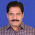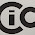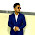## Friday, 31 August 2012

### Class 10 - Science - CH13 - Magnetic Effects of Electric Current

Magnetic Effects of Electric CurrentEarth's Magnetic Field (computer simulation) credits: wikipedia

MCQs, Q & A from CBSE Papers

Q1: The two poles in a magnet have
(a) equal pole strength.
(b) unequal pole strength.
(c)  can be same or different
(d) None of these

Q2:A magnet AB is broken into two pieces. What is the polarity of A, B, C and D?

(a) A, D: North & B,C: South
(b) A, B: North & C,D: South
(c) A,C: North & B,D: South
(d) A,D: South & B,C: North

Q3: Magnetic field lines:
(a) form closed curves
(b) cannot intersect
(c) are crowded near poles
(d) All of these

Q4: The correct field lines are:

Q5:Who had first observed the magnetic effect of an electric current first ?
(a) Faraday
(b) Oersted
(c) Volta
(d) Ampere

Q6(NCERT): The magnetic field inside a long straight solenoid-carrying current(a) is zero
(b) decreases as we move towards its end
(c) increases as we move towards its end
(d) is the same at all points

Q7: With the help of which law the direction of a magnetic field can be decided ?(a) Faraday's law
(b) Fleming's right hand rule.
(c) Right hand thumb rule
(d) Fleming's left hand rule.

Q8(NCERT): Which of the following property of a proton can change while it moves freely in a magnetic field? (There may be more than one correct answer.)
(a) mass
(b) speed
(c) velocity
(d) momentum

Q9(NCERT): Which of the following correctly describes the magnetic field near a long straight wire?(a) The field consists of straight lines perpendicular to the wire.
(b) The field consists of straight lines parallel to the wire.
(c) The field consists of radial lines originating from the wire.
(d) The field consists of concentric circles centred on the wire.

Q10: What is the field line of a magnetic field passing through the centre of current carrying circular ring ?(a) Circular
(b) Straight line
(c) Ellipse
(d) Magnetic field is zero at center.

Q11: Frequency of AC in India is
(a) 50 Hz
(b) 60 Hz
(c) 110 Hz
(d) 220 Hz

Q12: As we move away from a current carrying conductor, the spacing between the magnetic lines of force
(a) decreases
(b) increases
(c) remains at equal distances
(d) none of these

Q13(NCERT): A positively-charged particle (alpha-particle) projected towards west is deflected towards north by a magnetic field. The direction of magnetic field is
(a) towards south
(b) towards east
(c) downward
(d) upward

Q14: Whose magnetic field is like a magnetic field of a bar magnet?
(a) Current carrying wire
(b) Current carrying ring.
(c) Current carrying solenoid
(d) Current carrying rectangular loop

Q15(NCERT): A rectangular coil of copper wires is rotated in a magnetic field. The direction of the induced current changes once in each
(a) two revolutions
(b) one revolution
(c) half revolution
(d) one-fourth revolution

Q16: From which of the following case, the current in the loop will not be induced ?
(a) The loop is moved in the direction of the magnet.
(b) The magnet is moved in the direction of the loop.
(c) The loop and magnet are moved in the opposite direction with the same speed.
(d) The loop and magnet are moved in one direction with the same speed.

Q17(NCERT): The phenomenon of electromagnetic induction is

(a) the process of charging a body.
(b) the process of generating magnetic field due to a current passing through a coil.
(c) producing induced current in a coil due to relative motion between a magnet and the coil.
(d) the process of rotating a coil of an electric motor.

Q18(NCERT): The device used for producing electric current is called a
(a) generator.
(b) galvanometer.
(c) ammeter.
(d) motor.

Q19(NCERT): The essential difference between an AC generator and a DC generator is that
(a) AC generator has an electromagnet while a DC generator has permanent magnet.
(b) DC generator will generate a higher voltage.
(c) AC generator will generate a higher voltage.
(d) AC generator has slip rings while the DC generator has a commutator.

Q20(NCERT): At the time of short circuit, the current in the circuit
(a) reduces substantially.
(b) does not change.
(c) increases heavily.
(d) vary continuously.

Answers:
1: (a) equal pole strength.
2: (c) A,C: North & B,D: South
3: (d) All of these
4: (a)
5: (b) Oersted
6: (d) is the same at all points
7: (b) Fleming's right hand rule.
8: (c) velocity and (d) momentum
9: (d) The field consists of concentric circles centred on the wire.
10:  (b) Straight line
11: (a) 50 Hz
12: (b) increases
13: (d) upward [hint: use Fleming's left hand rule]
14: (c) Current carrying solenoid
15: (c) half revolution
16: (d) The loop and magnet are moved in one direction with the same speed.
17: (c) producing induced current in a coil due to relative motion between a magnet and the coil.

18: (a) generator.
19: (d) AC generator has slip rings while the DC generator has a commutator.
20: (c) increases heavily.

Q21(CBSE 2010): What does the direction of thumb indicate in the right-hand thumb rule?

Answer: Motion of the conductor.

Q22(CBSE ): Why does the acceleration of a bar magnet decreases while falling through a solenoid, connected to a closed circuit?

Answer: The induced current will exert an opposing force which will reduce the acceleration of falling bar magnet.

Q23(CBSE): Can two magnetic lines of force ever interact? Justify your answer.
or
Q(NCERT): Why don’t two magnetic lines of force intersect each other?

Answer: No. Two magnetic lines of force never interact. If they did, it would mean that at the point of intersection, the compass needle would point towards two directions, which is not possible.

Q24(CBSE 2010): What will be the frequency of an alternating current, if its direction changes after every 0.01 s?

Answer: If ac current changes its direct in 0.1s, it means it completes its cycle in 0.2s(0.1s+0.1s). Frequency = 1/Time period = 1/0.02 = 50Hz.

Q25(CBSE 2010): AB is a current carrying conductor in the plane of the paper as shown in figure. What are the directions of magnetic field produced by it at points P and Q? Given r1 > r2, where will the strength of the magnetic field be larger?

Answer: To know the direction of magnetic field produced by current passing through a conductor, we can apply 'Right Hand Thumb Rule' or Maxwell Corkscrew rule.
At point P, the magnetic field lines enter into the paper(screen). At point Q, the field lines are coming out of paper (screen)

Magnetic field follows inverse square law i.e. a magnetic field decreases inversely at a distance while moving away from the conducting wire.
Since r1 > r2, magnetic field is larger at Q than P.

Q26: Name two sources of direct current and alternating current.

Answer: DC source: dry cell, solar cell.
AC source: AC dynamo, turbines

Q27: What will happen to magnetic field due to a circular coil  carrying electric current, if the number of turns of the coil is doubled.

Answer: The magnetic field produced by a current-carrying wire at a given point depends directly on the current passing through it. If the number of turns in the circular coil is doubled, the field produced will also get doubled as that produced by a single turn. This is because the current in each circular turn has the same direction, and the field due to each turn then just adds up.

Q28(CBSE 2011): Why does a current carrying conductor experiences a force when it is placed in a magnetic field? State Fleming’s left hand rule.

(Note: What happens when two bar magnets are kept close to each other. They either repel or attract. It happens because the magnetic field lines of the two interact each other. The answer for the above question is also similar).

Answer: The current carrying conductor produce magnetic field. When this conductor is placed in a magnetic field, it experiences a force due to mutual interaction of these  two magnetic fields.Fleming's Left Hand Rule (credits:wikipedia)
Fleming's Left hand rule states, "When an electric current (I) flows in a wire, and an external magnetic field (B) is applied across that flow, the wire experiences a force (F) perpendicular both to that field and to the direction of the current flow."

It can be represented by the left hand. Stretch the thumb, forefinger and middle finger: of your left hand mutually perpendicular to each other such that the fore finger points in the direction of magnetic field and the middle finger in the direction of current, then the thumb will point in the direction of motion or the force acting on the conductor.

✏ Flemings' Left Hand rule for electric motors
✏ Flemings' Right Hand rule if for electric generators.

See also nice tutorial with beautiful graphics on Motors and Generator by Florida State University.

Q29(NCERT/CBSE 2010/2011): List the properties of magnetic lines of force.
or

Q(CBSE 2011): (i) What is the direction of magnetic field lines outside a bar-magnet ?
(ii) Why two magnetic field lines cannot intersect each other ?
(iii) What is indicated by crowding of magnetic field lines in a given region ?

Answer: Properties of magnetic lines of force are:
1. The imaginary lines which represent the direction of magnetic field, are known as magnetic lines of force.
2. Magnetic lines of force are closed curves. Outside the magnet their direction is from north pole to south pole and inside the magnet these are from south to north pole.
3. They neither have origin nor end.
4. These lines do not intersect, because if they do so then it would mean two value of magnetic field at a single point, which is not possible.
5. At the poles of the magnet the magnetic field is stronger because the lines of force there are
crowded together and away from the poles the magnetic field is weak.
Q30: When the current flows in a coil in anti-clockwise direction, what pole does it create?

Answer: North Pole

Q31(CBSE 2011): What is the advantage of a.c. over d.c.?

Answer:  The advantage of AC over DC is that electric power can be transmitted over long distances without much loss of energy.

Q32: What does MRI stands for?
Answer: Magnetic Resonance Imaging.

Q33: What colour conventions are used in domestic electrical wiring to indicate:
(i) live wire
(ii) neutral wire
(iii) earth wire

Answer:
(i) live wire - red
(ii) neutral wire - black
(iii) earth wire - green

Q34: State the principle on which an electric generator works?

Answer: It works on the principle of electromagnetic induction. It converts mechanical energy into electrical energy.

Q35: (i) What is a commutator?
(ii) In an electric motor which part act as commutator?
(iii) How does it help?

Answer:
(i) A device that reverses the direction of flow of current through a circuit is called a commutator.

(ii) In electric motors, the split ring acts as a commutator.

(iii) The reversal of current also reverses the direction of force acting on the two arms and generates a torque. Therefore the coil and the axle rotate half a turn more in the same direction. The reversing of the current is repeated at each half rotation, giving rise to a continuous rotation of the coil and to the axle.

Q36: (i) What is an armature? What is its advantage?
(ii) What are the advantages of electromagnet over permanent magnet?

Answer: The soft iron core, on which the coil is wound, plus the coils, is called an armature. It is used in electric motors to act as an electromagnet. The armature (electromagnet) enhances the power of the motor.
(ii) The advantages are:
1. You can turn the electromagnet by switching ON or OFF the current.
2. You can reverse the poles of the electromagnet by reversing the direction electric current flow in the coil.
3. The magnetic field strength can be changed by changing the amount of current in the coil.
4. An electromagnet can easily be made more powerful than a permanent magnet.
Q37: Define electromagnetic induction. What are the different ways to induce current in a coil?

Answer: The process, by which a changing magnetic field in a conductor induces a current in another conductor, is called electromagnetic induction.

We can induce current in a coil by applying any of the following:
(i) by moving it in a magnetic field
(ii) by changing the magnetic field around it.

Q38(HOTS): When a proton moves in a uniform magnetic field, why does the momentum change but its kinetic energy does not change?
(Revisit MCQ Q8 above).

Answer: The magnetic force exerted will be perpendicular to the direction of motion of the proton. As we know when when force acting is perpendicular to the direction of moving charge, work done will be zero. It means kinetic energy does not change. The force is able to change the direction (velocity) of the proton but not its speed (magnitude). Thus momentum and velocity changes.

Q39: Is magnetic field vector or scalar quantity?

Answer: Magnetic field is a vector quantity. It has both magnitude and direction.

Q40: Can a 5A fuse be used in wire carrying 15 A current? Why?

Answer:  Since the current withdrawn in the electric circuit is 15A, the fuse will blow frequently and remain ineffective.

Q41(CBSE 2011/NCERT): An electric oven of 2 kW power rating is operated in a domestic electric circuit that has a current rating of 5A. If the supply voltage is 220V, what result do you expect ? Explain.

Answer: Given,
Power of the oven (P)  = 2kW = 2 × 103 W
Voltage supplied (V) = 220V
Current (I) = ? A
Power = V × I
⇒ I = P/V = 2 × 103/ 220 = 9.09 A
Since the domestic electric circuit has a current rating of 5A, the flow of 9.09 A by the Oven exceeds the safe limit. Here, fuse will blow and break the circuit.

Q42: A current flows from the wire wound around an iron nail as shown in the figure below. Where is the North Pole and South Pole?

Answer: You may use right-hand rule i.e. if fingers of right hand curl in the direction of the current, thumb points towards the North pole. Top one will be South Pole and bottom face will be the North Pole.

Q43(CBSE): What are the different ways to strengthen an electromagnet?

Answer:
1. Can add second battery to increase the amount of current.
2. Add more number of turns in the coil.
In addition, an electromagnet also depends on the type of core used. Type of material, shape, size and winding pattern of the coil also control the shape and strength of the magnet field of an electromagnet.

Q44: A current of 5A is flowing through a conductor AB. Will the current be induced in the circular wire of give radius 1m?

Answer: Since a steady current of 5A is flowing through the conductor, no current or emf will be induced in the circular wire.

(In progress...)#### 16 comments:

1.2.3.Awsm. ........job keep it up😎☺☺☺😎

4.5.6.7.8.9.10.11.ahh very easy solved in 5 min

12.13.14.15.Maan gaye aap ko

16.Thank u it really helpful to me

We love to hear your thoughts about this post!